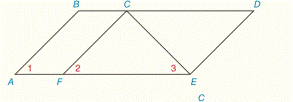Chapter 2.CR, Problem 33CR### Elementary Geometry for College St...

6th Edition
Daniel C. Alexander + 1 other
ISBN: 9781285195698

#### Solutions

Chapter
Section### Elementary Geometry for College St...

6th Edition
Daniel C. Alexander + 1 other
ISBN: 9781285195698
Textbook Problem
1 views

# Given: A B ¯ ∥ C F ¯ ∠ 2 ≅ ∠ 3 Prove: ∠ 1 ≅ ∠ 3To determine

To check:

The given statement.

Explanation

Given:

Line AB¯ is parallel to CF¯.

2 is congruent to 3.

Figure (1)

Property:

If two parallel lines are cut by a transversal, then a pair of corresponding angles is congruent.

Approach:

Line AB¯ is parallel to CF¯.

1 and 2 are corresponding congruent angles.

So,

12.

2 is congruent to 3.

23.

Use Transitive Property of congruence.

13

The proof for the given statement is shown in the following table,

 PROOF Statements Reasons 1. AB¯∥CF¯ 1. Given 2. ∠1≅∠2 2

### Still sussing out bartleby?

Check out a sample textbook solution.

See a sample solution

#### The Solution to Your Study Problems

Bartleby provides explanations to thousands of textbook problems written by our experts, many with advanced degrees!

Get Started

#### In Problems 45-51, solve each system of equations. 51.

Mathematical Applications for the Management, Life, and Social Sciences

#### In Exercises 107-120, factor each expression completely. 118. 8a2 2ab 6b2

Applied Calculus for the Managerial, Life, and Social Sciences: A Brief Approach

#### i=1n(4i2+i)= a) 4i=1n(i2+i) b) 4i=1ni2+i=1ni c) i=1n5i2 d) 4i=1ni2+4i=1ni

Study Guide for Stewart's Single Variable Calculus: Early Transcendentals, 8th

#### The graph at the right has equation:

Study Guide for Stewart's Multivariable Calculus, 8th